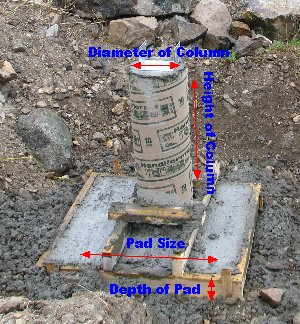Spike's Calculators

# Column + Pad Concrete Volume Calculator - MetricColumns need to be supported by footings. In some cases, this can be the same footings that support your foundation walls, but more often or not, they are on a separate footing, also known as a pad. For round columns, these pads usually are square.

To calculate the volume for a column plus pad, you need the diameter and height of the column plus the length of one of the sides and the depth of the pad.### Column & Pad Concrete Calculator

# of Columns + Pads: #
#Diameter of Column: mm
Height of Column: m

Cubic Meters:
Cubic Yards: yd³
Cubic Feet: ft³

#### Calculation

1. enter the number of columns and pads required
2. diameter of the column in millimetres
3. the height of the column(s)
4. length of one side of the pad in metres
5. depth of pad (thickness) in millimetres

#### Results

1. amount of concrete needed in cubic meters
2. converted into cubic yards
3. concrete needed in feet

##### Formula
column
`V = π * D² ÷ 4 * h * n`

`V = w² * d * n`

```where V = volume
π = 3.14159
D = diameter
h = height of the column
n = number of pads and columns
```
##### Example calculation
```column diameter = 254 millimetres = 0.254 metres
column height = 1.5 metres
pad size = 0.7 metres x 0.7 metres
thickness of pad = 254 millimetres = 0.254 metres
```

Calculating the volume of the columns using the above formula:
`3.14159 * 0.254² ÷  4 * 1.5 * 5`
`3.14159 * 0.064516 ÷  4 * 1.5 * 5 = 0.380`
`The columns have a volume of 0.380 cubic metres.`

Calculating the volume of the pads;
`0.7² * 0.254 * 5`
`0.49 * 0.254 * 5 = 0.6223`
`The volume of the five  pads is 0.6 cubic metres.`

`0.380  + 0.6223 = 1 .002`
`It will take 1 cubic metres of concrete to pour these five columns with pads.`
##### Conversions
```To convert cubic metres to cubic yards multiply cubic metres * 1.30795061931439
To convert cubic metres to cubic feet multiply cubic metres * 35.3146667214886```

### When Ordering Concrete

This concrete calculator will help you in estimating the amount of concrete needed for your project. The amount given as needed, does not include any waste. It is recommended, depending on the job you are doing, to add anywhere from an extra 4% to 10% to your concrete order to make sure you have enough concrete to finish the job. Ask when ordering the concrete. They will be able to give you a good idea of what is needed.